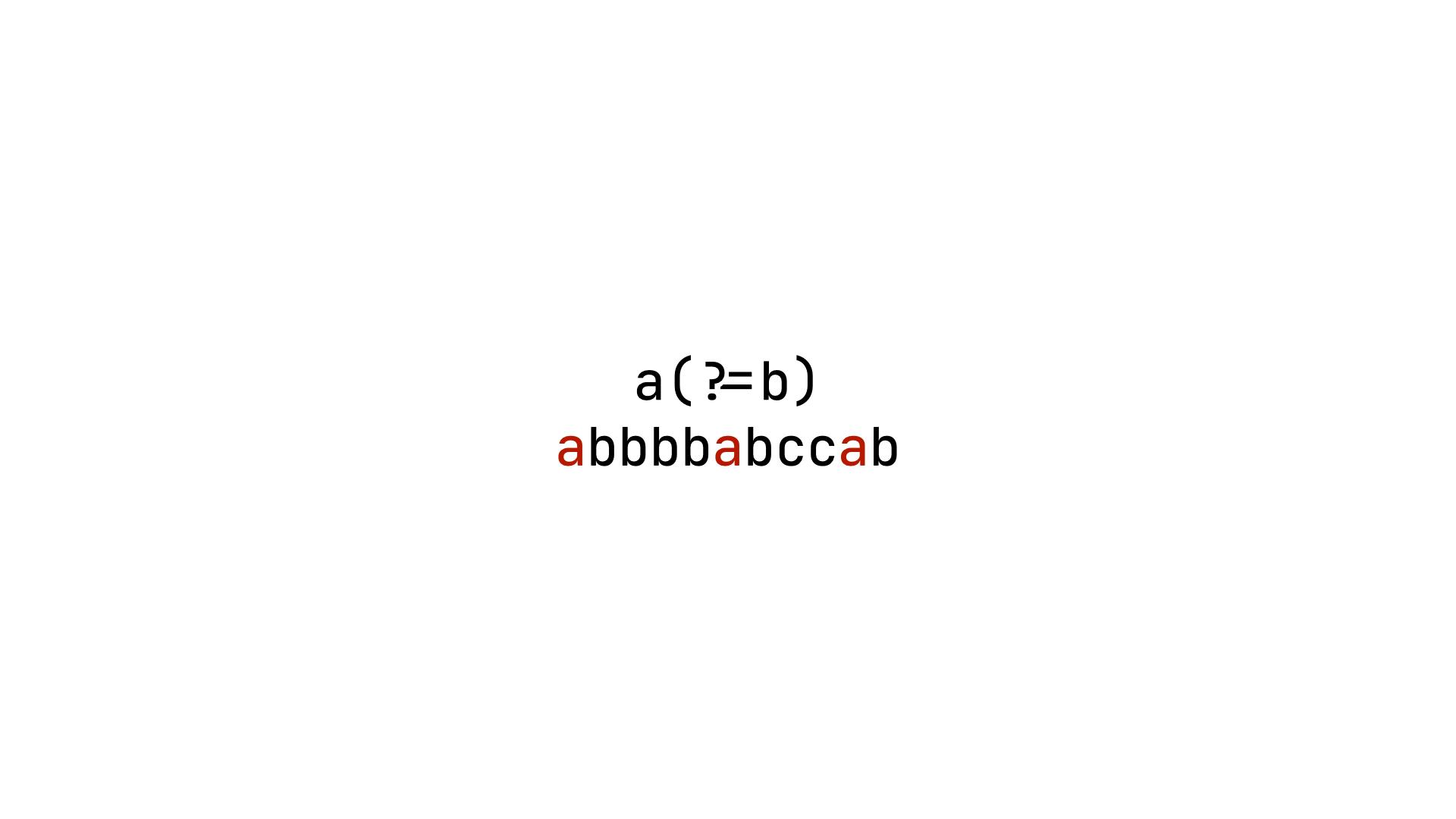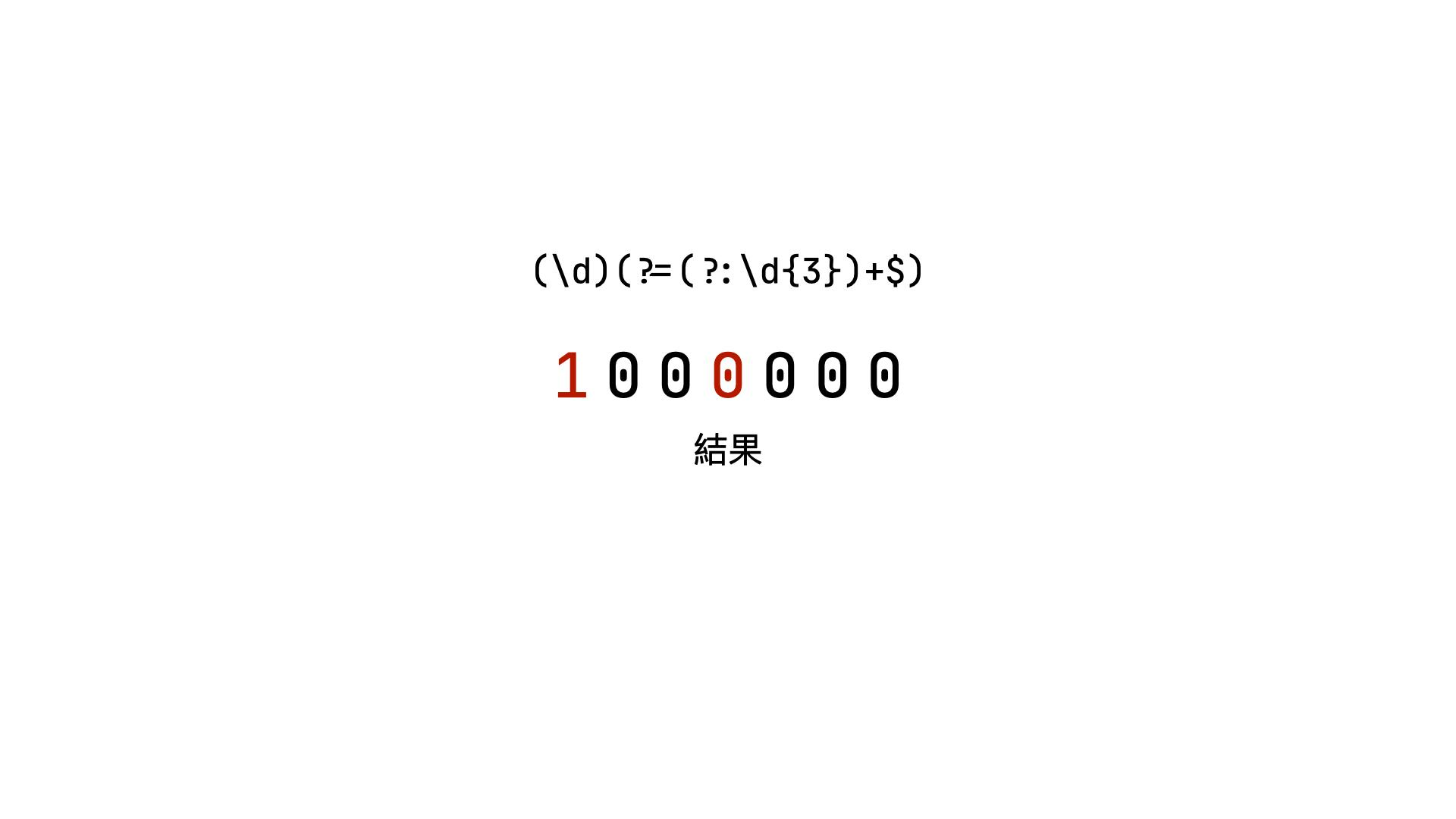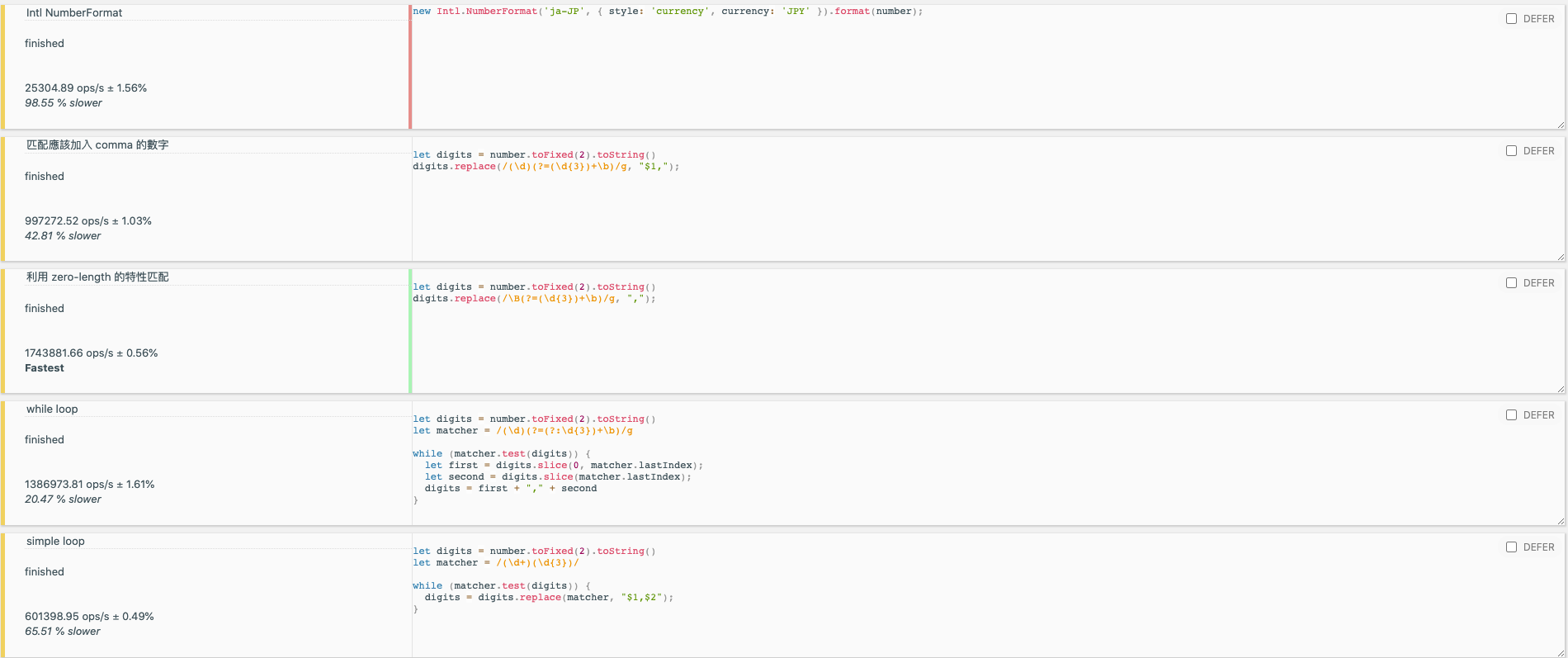# 魷型前端# 將數字加上 comma 的正規表達式說明

• 1234567 → 1,234,567
• 10000 → 10,000

• 透過 Intl.NumberFormat （老舊瀏覽器可能不支援，需要 polyfill）
• 透過正規表達式加上 .replace

const reg1 = /\B(?=(\d{3})+$)/ const reg2 = /(\d)(?=(\d{3})+$)/

## 前言（圖片中加入了 ?: 來表示不用將匹配結果放到 group 裡面，結果是一樣的）

"1000000".replace(/(\d)(?=(\d{3})+$)/g, "$1,"); // 注意這邊的 $1 這邊的 $1 很重要，因為我們要把匹配的字元也一起放進去，只有 , 的話會像這樣：,00,000

## 其他考量與方式

}NameOps/s
Zero-length /\B(?=(\d{3})+\b)/g1778943 ops/s fastest

while loop1371453 ops/s 22.91% slower
simple loop597173.88 ops/s 66.43% slower
Intl.NumberFormat25304.89 ops/s 98.55% slower